Next: Remove linear term heuristic. Up: Building additional iterators Previous: Transcendental Iterators.

#### Other special cases.

The technique used for polynomials can be extended to other cases, when we know how to solve some transcendental equations. In Maple, the function W(x)[2,4,3] is the solution of W(x)eW(x)=x. Hence many combinations of powers of x with ex and with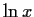are invertible. The resulting iterators have a lower number of occurrences of x on the rhs, and are considered better. Some of them are shown in table 4.

Table 4: Inverting expressions with the aid of the W(x) function
 equation iterator xaebx = F(x)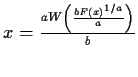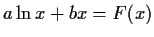xx=F(x)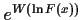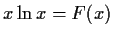eW(F(x))

Table: Iterators generated by solving a lhs involving, ex and x in terms of W(x)
 66644 random equations producing 3174 iterators method failures converged to a root 1.83% converged to a non-root .07% failed 98.17% diverged .80% average time .128 outside domain 1.14% time per root 6.985 too many iterations .20% iterator fails 0.00% iterators per equation .048 no iterators 97.79%

Table 5 shows the simulation results for lhs which can be resolved with the W(x) function. The iterators generated in this way are also very successful in producing roots, just as the non-linear polynomials. In this case however, only 2% of the equations can produce such iterators, and their relative usefulness is decreased. The W(x) function accepts a branch indicator, then as before we can compute an arbitrarily large number of iterators which will normally converge to different roots.Next: Remove linear term heuristic. Up: Building additional iterators Previous: Transcendental Iterators.
Gaston Gonnet
1998-07-08